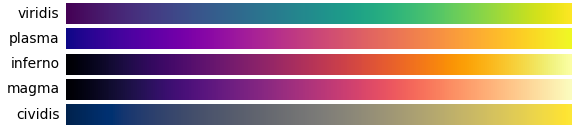## Matplotlib configuration

Images in BornAgain examples are generated using the Python library Matplotlib.

Default settings can be overridden by by function arguments, Matplotlib ressources, or environment variables.

### Plot invocation

When running BornAgain through Python sripts, Matplotlib is invoked either directly, or indirectly through BornAgain functions like run_and_plot. For direct invocation, see the Matplotlib documentation. Here we are concerned with indirect invocation through standard BornAgain plot functions.

The functions run_and_plot, plot_simulation_result, and a number of lower-level functions, are all implemented in the Python module plot_utils that is part of the bornagain module. These functions support the keyword arguments

• intensity_min,
• intensity_max,
• xlabel,
• ylabel,
• zlabel,
• cmap: color map, see below,
• aspect: aspect ratio, default is ‘auto’, other possible values are ‘equal’, or a number,
• noshow: if True, run Matplotlib in batch mode, without displaying a plot.

So if an example script contains the code line

    ba.run_and_plot()


then the plot can be modified through keyword arguments like

    ba.run_and_plot(cmap='jet', intensity_min=2e-8, intensity_max=2)


### Matplotlib ressources

Matplotlib default settings can be changed through ressource arguments or ressource files. See the Matplotlib customizing tutorial for an introduction to ressources; see in particular the section on matplotlibrc ressource files. Note, however, that the color map (cmap) ressource setting is ignored when Matplotlib is used via BornAgain plot functions.

### Environment variables

BornAgain supports two specific environment variables:

• NOSHOW, to prevent plot routines from opening a plot window; for use in tests and other batch processing;
• CMAP, the default color map, see below.

### Color map

Whenever plotting is done through plot_simulation_result, or through one of the other functions from plot_utils.py, BornAgain imposes its default color map “inferno”. Inferno is one of the five “perceptually uniform sequential” color maps recommended in the Matplotlib color maps tutorial.Note that our choice of “inferno” is hard-coded, and overrides the CMAP environment variable. It can however be overridden by a keyword argument, like cmap='jet'.